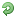# Thread: How to get sheet count of an excel workbook in QTP?

1. ## How to get sheet count of an excel workbook in QTP?

I want to get the sheet count of an excel workbook in QTP.

Let me know the different methods of doing it.Reply With Quote

2. ## Re: How to get sheet count of an excel workbook in QTP?

hai friend,

Datatable.ImportSheet "C:\Documents and settings\specify your user name here\Desktop\test.xls",1,1
sheetcount = DataTable.GetSheetCount
Msgbox sheetcount

To get sheet count what I have done here is I have imported an excel sheet named(test.xls) and then counted the number of used sheet in that excel workbook.

Note: This method is used to find the total number of sheets in the run-time Data Table

Thanks
DeepaReply With Quote

3. ##Re: How to get sheet count of an excel workbook in QTP?

Hi Deepa

how to get sheet count of external excel sheet without import the excel sheet?Reply With Quote

4. ## Re: How to get sheet count of an excel workbook in QTP?

Hi,

Try the below code which uses the method of creating an Object of Excel -

Dim objExcel
Dim sPath

Set sPath = "<>"

Set objExcel = CreateObject("Excel.Application")
Set objWorkbooks = objExcel.Workbooks.Open(sPath)

nWorkSheetCount = objExcel.Worksheets.count

msgbox "There are a total of" & nWorkSheetCount & "worksheets"

Cheers,Reply With Quote

5. ## Re: How to get sheet count of an excel workbook in QTP?

Hi friend,

The code should be as follows

Dim objExcel
Dim sPath
sPath="C:\New Folder\Sample"
Set objExcel = CreateObject("Excel.Application")
Set objWorkbooks = objExcel.Workbooks.Open(sPath)
nWorkSheetCount = objExcel.Worksheets.count
msgbox "There are a total of" &nWorkSheetCount &"worksheets"

Method 2

Set objExcel = CreateObject("Excel.Application")
Set objWorkbook = objExcel.Workbooks.Open _
("C:\New Folder\Sample")
objExcel.visible="True"
SheetCount = objExcel.Worksheets.count
msgbox SheetCount

Thanks,
DeepaReply With Quote

6. ## Re: How to get sheet count of an excel workbook in QTP?

What is the difference between Workbook.Worksheets and Workbook.Sheets?Reply With Quote

7.

####Posting Permissions

• You may not post new threads
• You may not post replies
• You may not post attachments
• You may not edit your posts
•